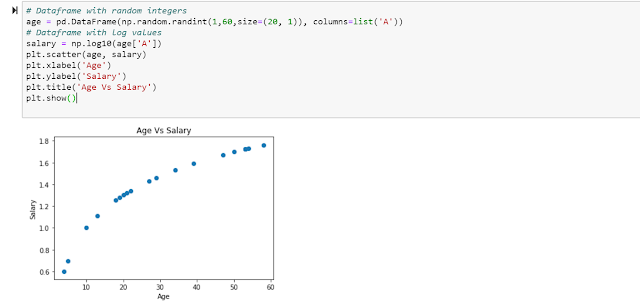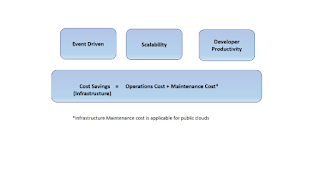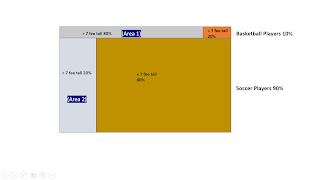## Posts

Showing posts from 2019

### Quick Introduction to MatplotlibOverview:In Datascience and Machine learning, plots and graphs play a very important role. To understand the data and its characteristics, it is very important to plot the data and make sense out of it. Matplotlib is a powerful Python library which is very handy in building effective plots. We will take a look at a brief intro to Matplotlib.
Scatter Plot:Scatter Plot plots two variables one each along X and Y axis and provides a view of the relationship or correlation between the two variables. Let us create two variables, where one variable has an exponential relationship with the other (i.e logarithmic relationship).
Let us hypothetically assume that age and salary are related exponentially. So as age increases, salary increases exponentially. Let us create two dataframes with this in mind and plot them. Note how we added labels for X and Y axis and also a title for the plot.

Line Plot:We can also plot two variables and draw a line to reflect the relationship between them. Matplotlib w…

### Quick Introduction to Pandas LibraryOverview:Pandas is a python library which provides very powerful tools to perform complex data manipulations and analysis. In this article, few of the commonly used operations are explained.
A common datastructure is a 'Dataframe'. Dataframe is a matrix type of datastructure with easy access to rows and columns.
Creation of Dataframe:

Accessing data from Dataframe:Data in Dataframe could be accessed in various ways.

Data can also be accessed by slicing the dataframe instead of loops. It is the most efficient way of accessing data.

Creating Dataframe with Index:We can create Dataframe with an index. Let us create a dataframe with date as index.

As we can see from above, the values are filled as NaN (Not a Number). We can replace NaN with zero using fillna() function.

### Quick Introduction to Numpy LibraryOverview:Numpy is a popular library in Python, which is used in Machine Learning and Statistical analysis. This article provides some of the commonly used operations in Numpy. Numpy array operations are efficient and fast compared to normal array operations.

Jupyter Notebook:The code is run using Jupyter Notebook and the relevant snapshots are provided. Note: Numpy could be imported as below:import numpy as np
Array Operations:Basic Operations:Array could be created using np.array(). Shape property provides a tuple of number of rows and columns. We can apply various mathematics functions like mean, median etc on the array.

Comparison in Array: We can apply various techniques to compare one row against other, which would be required when we perform computational analysis.

Array Data Retrieval: We can retrieve data from an array, in terms of rows, columns. We can also, retrieve specific rows or columns or exclude specific rows or columns.

Max Min Operations: We can find out various maximum and m…

### Serverless Technology SpectrumIn this article, I am going to introduce you to Serverless technology in various flavors along with a usecase and its benefits.
What is Serverless? First of all, Serverless does not mean 'No Servers!!' (It is a misnomer). There are various definitions for Serverless, we will try to look at what Serverless means in various contexts.
Let me illustrate through an analogy. Analogy: Let us consider a scenario where, there are a number of doctors (dentists, chiropractors etc) who want to serve their patient, but they do not want to invest in real estate for the clinics, equipment and also do not want the burden of managing/repairing their equipment. To cater to their needs, an investor comes forward and builds a huge building and also procures all the necessary tools and instruments needed by various doctors. When a patient arrives, the doctor will be provided any vacant room and the room will be quickly setup with all the instruments. Once the consultation completes, the room is a…

### Terraform Part 2

In the previous post 'Intro to Terraform', I provided an introduction to Terraform. In this post, I will be digging a little bit deeper into how Terraform could be used in real world usecases.

How to use organize Terraform files?We can organize Terraform files so that, each component which we would provision would go into its own .tf file. That way, we can have a modularized approach.
provider.tfThis file would have the provider configuration details, so that any change to provider related config could be done in one place.

vars.tfThis file would have the variables used by every different file. We can variabilize different parts of our infrastructure configuration, like for eg, the CIDR ranges which will be used in VPC and subnet. This way, we can parameterize our provisioning code.
One tf file per Component:We can have one .tf file per component, for instance, a vpc.tf file, a subnet.tf file etc. This way we can isolate changes.
Data Selector:Another important feature which we can…

### Bayes TheoremIn this article, let us try to understand Bayes Theorem.
This article has been inspired by the two videos provided in References section. The illustrations used here are my own.

Bayes theorem helps us draw inferences from data. It also challenges our beliefs which could be often biased.
Let us say that we came across a group of athletes from many countries with the athletes from top 2 or 3 popular sports from those countries.
We will consider a group of athletes who play either soccer or basketball.

The height of one of the athletes is more than 7 feet. What do you think this athlete plays?
Soccer or Basketball.

Our intuition definitely says that he must be playing basketball.
Now, let us do the math.

The most popular sport played across the globe is soccer (No offense to Basketball!). Let us say we have total of 100 athletes and 90% of them play soccer and 10% play basketball.
That means 90 athletes play soccer.

Now let us see how many of soccer players are more than 7 feet tall. We might hav…